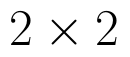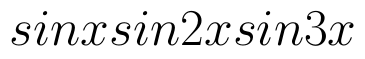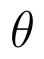Select Page

# MCQ Matrices CBSE Maths 12 Science Answers in English

MCQ Matrices CBSE Maths 12 Science Answers in English to enable students to get Answers in a narrative video format for the specific question.

Expert Teacher provides MCQ Matrices CBSE Maths 12 Science Answers through Video Answers in English language. This video solution will be useful for students to understand how to write an answer in exam in order to score more marks. This teacher uses a narrative style for a question from Matrices not only to explain the proper method of answering question, but deriving right answer too.

Please find the question below and view the Answer in a narrative video format.

Question:

## Similar Questions from CBSE, 12th Science, Maths, Matrices

Question 1 : Find the transpose of the matrix:. (View Answer Video)

Question 2 :  Find the value of y, if(View Answer Video)

Question 3 : Find the value of Y, ifand(View Answer Video)

Question 4 : If for any squarematrix A,then write the value of |A|. (View Answer Video)

Question 5 : Find the transpose of the matrix:. (View Answer Video)

### Integrals

Question 1 : Find the integral of the function. (View Answer Video)

Question 2 : Find:. (View Answer Video)

Question 3 : Find the integral of the function. (View Answer Video)

Question 4 : Evaluate :(View Answer Video)

Question 5 : Find :(View Answer Video)

### Three Dimensional Geometry

Question 1 : Find the equation of the plane passing through the line of intersection of the planesandwhich is perpendicular to the plane. (View Answer Video)

Question 2 : If a line marks anglesandwith x, y and z-axis respectively, whereis acute, then find. (View Answer Video)

Question 3 : Write the vector equation of the plane passing through the point (a, b, c) and parallel to the plane(View Answer Video)

Question 4 : Find the equation of the plane with intercept 3 on the y-axis and parallel to ZOX plane. (View Answer Video)

Question 5 : What is the distance of the point (p, q, r) from the x-axis ? (View Answer Video)

### Relations and Functions

Question 1 : Let * be the binary operation on N given by a * b = LCM of a and b. Find 5 * 7. (View Answer Video)

Question 2 : Let A = {1, 2, 3}. Then, number of relations containing (1, 2) and (1, 3) which are reflexive and symmetric but not transitive is, (View Answer Video)

Question 3 : If f(x) = |x| and g(x) = | 5x – 2 |. Then, fog = _____. (View Answer Video)

Question 4 : Functionsare defined respectively, by, find. (View Answer Video)

Question 5 :  Let R be the relation on the set {1, 2, 3, 4} given by R = {(1, 2), (2, 2), (1, 1), (4, 4), (1, 3), (3,3), (3,2)}. then R is, (View Answer Video)